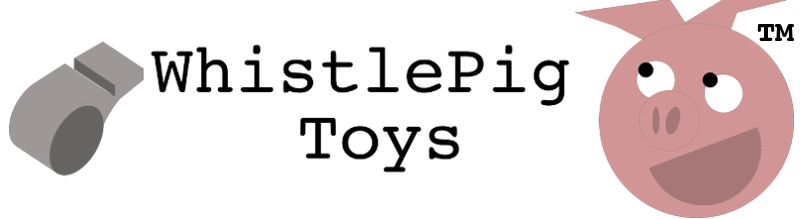# Center of Gravity, or Center of Mass?

One of my favorite things to do when I draw plans for a model airplane is to mark the CG symbol. The CG is the heart and soul of an airplane. It is the point around which everything revolves, it is the sum of all the parts, it is the expression of compromises and the solution to the interlocking problems of weight, size, and strength.I even like the sound of the initials: "C.G." Of course, having been trained in physics, I know that perhaps the more proper term is "Center of Mass". But C.M. doesn't really roll off the tongue nicely, and I miss the tradition of calling it the Center of Gravity. You might be asking yourself, "what's in a name? Aren't they really the same thing?" Or you might be wondering "Why not say Center of Mass since that's the Right Thing? It's like the difference between Weight and Mass right?"

So what are these things, and which term is more correct? When we talk about a "center" in this context, what we really mean is the "centroid", or the point upon which all forces act directly, with zero torque. It's the balance point. A centroid can be found for any forces you care to consider. On an airplane, there's a centroid of forces due to moving air, and a centroid due to gravity acting on the mass of the airplane, and a centroid due to the thrust of the engine. The C.G. is the centroid of forces acting due to gravity. It's pretty easy to find. All you need to know the the location of each mass relative to some reference location, (known as a datum), and the weight of each item. Multiply the weight times the distance of each part, add all those values together, and divide by the weight of the airplane. The answer is the distance to the CG from the reference.

Here's an example. Lets find the center of gravity of rocket as it stands on its fins:

``````Fuel Tank weight:   90kg * 9.81m/sec^2 = 882.9 Newtons
Engine weight:      60kg * 9.81m/sec^2 = 588.6 Newtons

Weight  Distance from ground     Moment
Fuel Tank   882.9 N     100cm        88290 N*cm
Engine      588.6 N     200cm       117720 N*cm
-----------------------------------------------------------
Total:      1471.9 N            206010 N*cm

CG = 206010N*cm / 1471.9 N = 140cm from ground
``````

If we ignore gravity, we can do it again:

``````        Mass    Distance from ground     Moment
Fuel Tank   90 kg       100cm       9000 kg*cm
Engine      60 kg       200cm       12000 kg*cm
-----------------------------------------------------------
Total:      150kg               21000 kg*cm

CM = 21000kg*cm/150kg = 140cm from ground
``````

So would seem to also be the distance to the CM. It's not, quite. Why? Because the acceleration due to gravity isn't quite constant. The well known value of 9.81m/sec^2 (or 32.2 ft/sec^2 in english units) is an average value at the surface of the earth. In fact, it varies with the inverse square of distance from the center of the earth.

``````g = G * mearth / (r^2)
``````

(where big G is the universal gravitational constant).

You can see what will happen to our rocket's center of gravity. The fuel tank, being further from the ground, is also further from the center of the earth, so it's weight will be slightly less. Its moment will be slightly less, and the CG will be closer to the ground. The center of mass remains unchanged.

Of course, in a practical sense this is a tiny change, but spacecraft can use this fact to orient themselves in space. Since practically the only forces acting on a spacecraft are gravitation, even a slight difference between the center of gravity and the center of mass will cause the spacecraft to rotate so that the center of gravity is closest to the earth.

Why? Because the center of mass is the point where a single applied force causes no rotation.

Since the C.G. and the C.M. are not the same, the force due to gravity is not applied at the C.M., and a rotational acceleration will occur.

You might ask why the Center of Mass has this property that a force applied to it causes no rotation. Here goes:

Newton's second law tells us that any force applied a mass causes an acceleration:

``````F = m * a
``````

or:

``````a = F / m
``````

Suppose we apply a force to our rocket in such a way that it does not rotate. All parts of it must then accelerate together.

We know our rocket masses 150 kg. Let's apply a force of 1500 Newtons to it. The rocket will accelerate at:

``````a = 1500N / 150kg = 10m/sec^2
``````

But where is the point we must apply this force so that it does not rotate? A lack of rotation means that the moments of all the forces due to the acceleration add up to zero - that is, they must balance.

``````Force on Fuel Tank: 90kg * 10 m/sec^2 = 900 Newtons
Force on Engine:    60kg * 10 m/sec^2 = 600 Newtons

Force   Distance from ground     Moment
Fuel Tank   900 N       100cm        90000 N*cm
Engine      600 N       200cm       120000 N*cm
-----------------------------------------------------------
Total:      1500 N          210000 N*cm

Balance point = C.M.  = 210000 N*cm / 1500N = 140cm from ground
``````

How about that - we found the CM.

So which one is more correct for an airplane, the C.M. or the C.G.?

One thing to consider is so obvious it's easy to forget: without gravity, there would be no need for airplanes! The force due to gravity (weight) is what flight must overcome, so we really can't pretend there's no gravity.

The inertial forces due to accelerations and rotations are crucial to understanding stability in airplanes, so the center of mass is just as important.

Conveniently, both the C.G. and the C.M. are very close together, so we save a little work. And I'd argue it's safe to use the term that makes you smile.